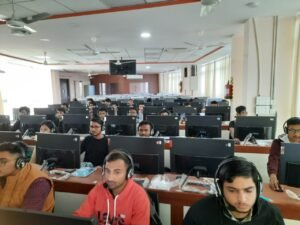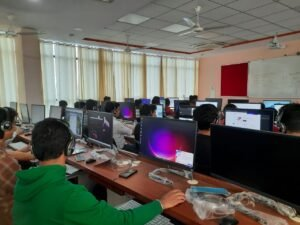Program Objectives: C is a powerful general-purpose programming language. The importance of computer programming in the modern day to day life and automotive industry is paramount and growing. So, the aim of this program is to make students can gain broad perspective about the uses of computer programming in engineering industry and to improve their analytical and logical thinking. Moreover, the use of C programming language is to create many types of applications and operating systems such as Windows, and other complicated programs such as the Oracle database, Python interpreter, and games etc.

Procedure of Program: To impart the basic knowledge about C programming such as, concepts of arrays, pointers, files, union, structures, conditional branching, iteration and recursion. Students will be able to develop logics which will help them to create programs, applications in C programming language. Through writing program on distinct topics, their logical and analytical approach will enhance. Additionally, by learning the fundamentals of C programming, students will be able to learn other programming languages with ease.

 S. No. Title of Lab Experiments 1. Write a program to find divisor or factorial of a given number. 2. Write a program to find sum of a geometric series 3. Write a recursive program for tower of Hanoi problem 4. Write a recursive program to print the first m Fibonacci number 5. Write a menu driven program for matrices to do the following operation depending on whether the operation requires one or two matrices a.      Addition of two matrices b.     Subtraction of two matrices c.      Finding upper and lower triangular matrices d.     Transpose of a matrix e.      Product of two matrices. 6. Write a program to copy one file to other, use command line arguments. 7. An array of record contains information of managers and workers of a company. Print all the data of managers and workers in separate files. 8. Write a program to perform the following operators on Strings without using String functions a.      To find the Length of String. b.     To concatenate two strings. c.      To find Reverse of a string. d.     To copy one string to another string. 9. Write a Program to store records of a student in student file. The data must be stored using Binary File. Read the record stored in “Student.txt” file in Binary code.Edit the record stored in Binary File.Append a record in the Student file. 10. Write a programmed to count the no of Lowercase, Uppercase numbers and special Characters presents in the contents of text File.

CONTENT BEYOND SYLLABUS

 S.No Name of Experiment 1. Write a C program to compute the monthly pay of 100 employees using each employee’s name, basic pay. The DA is computed as 52% of the basic pay. Gross-salary (basic pay + DA). Print the employees name and gross salary 2 2’s complement of a number is obtained by scanning it from right to left and complementing all the bits after the first appearance of a 1. Thus 2’s complement of 11100 is 00100. Write a C program to find the 2’s complement of a binary number. 3 Write a C program that uses functions and structures to perform the following operations: i) Reading a complex numberii) Writing a complex numberiii) Addition of two complex numbersiv) Multiplication of two complex numbers

Suggested Books

Text Books:

[T1] Programming in ANSI C by E. Balguruswamy, Tata Mc-Graw.

[T2] Programming With C, Schaum Series.

Reference Books:

[R1] Let Us C, Yashavant Kanetkar, Fifth Edition, 2021.

[R2] The ‘C’ programming language by Kernighan and Ritchie, Prentice Hall

[R3] Programming Logic & Design by Tony Gaddis, Pearson, 2nd Ed. 2016.

[R4] How to solve it by Computer by R.G. Dromey, Pearson Education